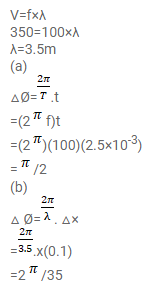# A sound wave frequency 100 Hz is travelling in air.Question:

A sound wave frequency $100 \mathrm{~Hz}$ is travelling in air. The speed of sound in air is $350 \mathrm{~m} \mathrm{~s}^{-1}$.

(a) By how much is the phase changed at a given point in $2.5 \mathrm{~ms}$ ?

(b) What is the phase difference at a given instant between two points separated by a distance of $10.0 \mathrm{~cm}$ along the direction of propagation?

Solution: